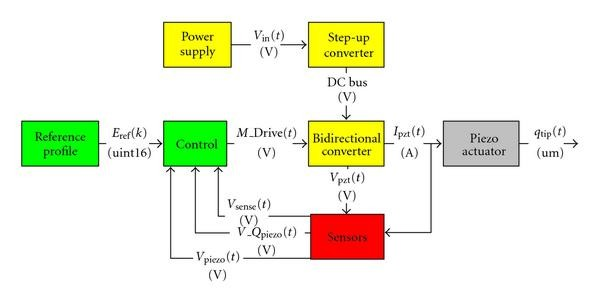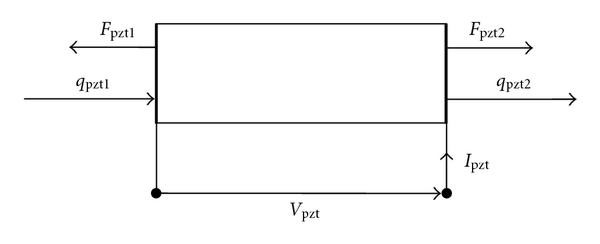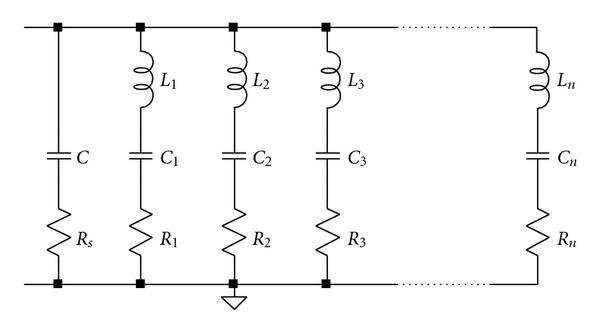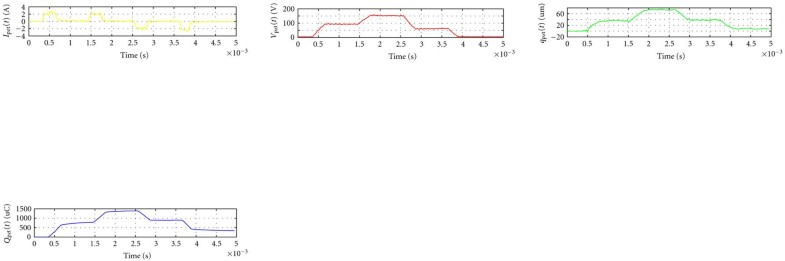EURASIP Journal on Embedded Systems

, 2010:578591

# Model and Design of a Power Driver for Piezoelectric Stack Actuators

Open Access
Research Article
Part of the following topical collections:
1. Design Methodologies and Innovative Architectures for Mixed-Signal Embedded Systems

## Abstract

A power driver has been developed to control piezoelectric stack actuators used in automotive application. An FEM model of the actuator has been implemented starting from experimental characterization of the stack and mechanical and piezoelectric parameters. Experimental results are reported to show a correct piezoelectric actuator driving method and the possibility to obtain a sensorless positioning control.

## Keywords

Piezoelectric Actuator Needle Position Electrical Equivalent Circuit Equivalent Capacitance Parallel Branch
These keywords were added by machine and not by the authors. This process is experimental and the keywords may be updated as the learning algorithm improves.

## 1. Introduction

In linear piezoelectricity, the equations of linear elasticity are coupled to the charge equation of electrostatics by means of the piezoelectric constants. However, the electric variables are not purely static, but only quasistatic, because of the coupling to the dynamic mechanical equations.

The aim is to obtain an analytical model in order to study the displacement of the free end of the stack and the system dynamics. To improve the efficiency of the transconductance amplifier employed, a switching bidirectional converter has been developed to correctly control the tip displacement of the stack and to recover the energy stored in the actuator during the discharge phase; this solution minimizes the required PCB area and reduces the input power. The control strategy is chosen in order to drive the piezoelectric actuator in charge for accurate tip displacement estimation and reduction of the intrinsic hysteresis of material. The complete system can be described in Figure 1.Figure 1 Schematic diagram of the proposed control architectures.

## 2. Piezoelectric Stack Actuator Model

Hysteresis is observable in open loop operation; it can be reduced by charge control (Figure 2) and virtually eliminated by closed loop operation.Figure 2 Piezoelectric hysteresis behaviour with voltage and charge driving strategies.

There are fundamentally two different driving methods for piezoelectric actuators:

Piezoelectric stack displacement depends on the charge stored on it; so using a charge feedback amplifier, it is possible to correctly drive the actuator. Considering the piezoelectric actuator as a 4-port element, for charge driving techniques, inputs are charge and external force (if present) and the outputs are tip displacement and voltage drops on it (see Figure 3). Using instead voltage driving techniques, inputs are voltage and external force and outputs are charge and tip displacement. For piezoelectric stack, we can create an FEM model that is useful to study the displacements of the free end of the stack and the system dynamics.Figure 3 Schematic representation of a piezoelectric element.

This model does not consider damping in a first stage: this effect is introduced in a second stage taking into account the experimental response of the piezoelectric actuator in resonance conditions. Including the mechanical dynamic behavior, this equivalent model can be easily implemented in electronics simulation software.

For a piezoelectric material, the equations we need are clearly standardized in the document ANSI/IEEE STD 176-1987, which expresses piezoelectric equations and physical constants in SI units.

The constitutive equations of a piezoelectric material at a microscopically level are the following:

or in an equivalent form:

The meaning of each symbol conventionally used in previous expressions is the following:

The relations that regulate the behavior of the entire piezoelectric actuator are derived from a classic FEM approach based on the standard defined equations.

The core of the actuator is a stack of piezoelectric layers. Electrically speaking, the layers are in parallel, while from a mechanical point of view they are in series. Applying a voltage to the electrical leads of the stack will cause a displacement of the mechanical sides and a force on the faces that are respectively the sum of displacements and forces of each layer.

Once defined the potential and kinetic energy associated to the element, it is possible to obtain the two electromechanical equations that regulate the behavior of the piezoelectric element. If we consider charge driving, the equations of the stack are

While using voltage driving, the equations are

Mechanical and electrical dissipations are not considered during this step. The meaning of each symbol conventionally used in previous expressions is the following:

(iv) is the electromechanical coupling matrix,

It is possible to transform the nodal equations into modal equations in order to consider each mode independent from the others. Considering only the resonances in the frequency range between 10 Hz and 100 kHz, the model has been reduced to the first five modes. The goal is to obtain electrical impedance in order to know the load to be applied to the power driver.

Reducing the mechanical model, it is possible to convert it into a frequency domain as shown in (5) as equivalent admittance.

Performing a low-frequency analysis (), the inertial properties of each mechanical parallel branches can be neglected, while elastic contributes are summed to the electrical capacitance:

So, the quantity corresponds to the equivalent low-frequency admittance. Doing then a first-mode analysis, we should detract the first-mode quantity from equivalent low-frequency capacitance:

Impedance poles are admittance zeros; so in order to find impedance natural frequencies, admittance's numerator is imposed equal to zero.

Keeping apart the parameter in the gradient of the curve, it is possible to impose equal to zero just on the part in brackets:

First zero's pulsation is

where are pulsations of admittance antiresonances, which are in correspondence to the impedance resonances. From experimental tests on the piezoelectric stack or from FEM analysis, it is possible to obtain the value of the first antiresonance pulsation (which also is the first admittance resonance value) . Substituting this value in the last expression, the first residue is

In this way, the admittance associated to the first natural frequency is

Keeping then in consideration all the successive modes, it is possible to extend the matter in order to determinate all the successive residues. For the first modes, impedance can be written as

## 3. Introduction of Mechanical and Electrical Losses

In order to obtain a model closer to the reality, it is necessary to introduce a modal damping element into each resonance term of the transfer function. It is important to keep in consideration a resistance in series with the piezo capacitance, which models the electrical losses due to electrical connections. Including damping, the resulting admittance is

Damping can be considered just near natural frequencies relative to the branch, while at each other frequency can be neglected.

## 4. Definition of the Equivalent Electrical Circuit Parameters

From (15), it is clear that the electric equivalent circuit of the mechanical properties is made up by n parallel branches to piezoelectric equivalent capacitor C. Each branch is made up by a capacitor and an inductor (in first approximation losses are neglected), so the physical admittance of each parallel branch of the electrical equivalent circuit is

Starting from the admittance obtained from modal analysis, it is possible to rewrite the last expression, in order to do a term-by-term comparison and determinate the parameters for the electrical equivalent circuit.

Our analysis is limited to the first five most important modes, starting from the admittance related to the first mode:

A term-by-term comparison with the first parallel branch parametric admittance leads to

The following relations can be used in order to define lumped parameters values for the electrical equivalent circuit:

All the relations before can be used to evaluate the parameters relative to the first branch and can be extended to each successive branch of the electrical equivalent circuit:

Residues value can be used to characterize the inductive and the capacitive elements of the electrical equivalent implementation, while resistance value is determined by comparison with experimental data obtained on piezo stack sample.

## 5. Electrical Equivalent

The admittance can be represented using the electrical equivalent circuit in Figure 4, where parallel branches correspond to the first 5 modes of the stack (electrical equivalent of mechanical modes).Figure 4 Electrical equivalent circuit for piezoelectric actuator model.Figure 5 FEM equivalent model and experimental measurements comparison.

A comparison between the experimental impedance and the result of electrical equivalent model is showed in Figure 5.

The electrical admittance is a good approximation of the piezo stack electrical behavior, so it will be used as a "reference load" for power driver design.

## 6. Power Driver Design

Injector controller is aimed at the control of the fuel quantity injected in the combustion chamber of the cylinders. This can be done by performing a position control on the injector needle.

Unfortunately, no velocity, position, or force measure can be performed inside the injector, neither on the piezoelectric stack nor on the leverage.

So, in order to estimate the injector needle position, it is important to measure a related quantity. The constitutive equations of a piezoelectric stack showed that the needle position is strictly related to the electrical charge loaded in the equivalent capacitance of the injector itself. A direct charge measurement is also possible but it is an intrusive technique. Nevertheless, loaded charge is proportional to the current transferred to the piezo stack by the power driver.

The implemented control is based on two different feedback loops:

(1)an inner loop which controls the injector current,

(2)an outer loop which controls the charge stored in the injector.

Hence from the charge stored in the piezo equivalent capacitance, it is possible to estimate the needle position.

From the models above, it is possible to consider the piezo stack as a capacitive load. A good method to drive a capacitive load is to use a current generator driven by an external signal: the simplest way to implement this type of current generator is to use a transconductance amplifier driven by a PWM signal (see Figure 6).Figure 6 Schematic architecture of the proposed driving stage.Figure 7 Schematic circuit of the bidirectional converter.

A good solution to obtain a high-efficiency amplifier is to use a class D amplifier with current mode control in order to have a quasi-ideal current generator .

The chosen topology is based on a synchronous bidirectional Buck Converter operating in a Continuous Conduction Mode (CCM) in order to reduce current stresses on electronic components, where the two transistors are driven in a complementary way. To obtain the complete discharge of the piezoelectric equivalent capacitance, a dissipative section (braking transistor) has been added.

The most important physical quantity for a piezoelectric actuator is the charge stored in the equivalent capacitance which depends on temperature. To cover all the possible driving techniques, the current flow to/from the actuator and the related voltage on it must be carefully measured and controlled. The basic converter scheme is proposed in Figure 7.

## 7. Power Driver Control

Power driver control is based on an inner current loop and an outer energy loop that controls the equivalent energy of the actuator. The inner loop is a hysteretic current mode controller with quasi constant frequency that solves the problem of sub harmonic instability, guarantees a quasi constant switching frequency, and reduces the inductance current ripple (Figure 8).Figure 8 Power driver control architecture with current and frequency control loops.

The hysteretic "window" is adjusted by varying the valley current reference value starting from the difference between reference switching periods and measured ones.

Starting from a hysteretic width initial value, the control system measures the switching period, and using a frequency feedback loop, the integral regulator determines the hysteresis variation in order to maintain the frequency quasi constant .

The outer charge/energy loop (Figure 9) is a classic PI control law with anti-windup that follows the reference profile.Figure 9 Power driver control architecture with charge/energy loop.

## 8. Implementation

The proposed system is implemented using a DSP/FPGA based prototyping control platform developed at Mechatronics Lab. In Figure 10, it is possible to see the power board with the bidirectional converter (on the left) and the FPGA controller board (on the right) between dummy loads (top) and the piezoelectric injector (bottom).Figure 10 The experimental set-up of the proposed FPGA controlled power driver with dummy loads and a piezoelectric actuator connected.

The power board is also provided with a boost DC/DC converter  also controlled from the same FPGA device in order to make the system compliant with automotive applications where the standard 12 V power source is not useful to drive the piezoelectric actuators.

The entire FPGA project takes up to 12 k logic elements (on a maximum of about 33 k available on the ALTERA Cyclone II device used in the application) divided into 18 main entities; the implemented code uses about 150 kbits of the memory embedded on the FPGA and 4 DSP elements for the fast fixed point multiplications. The main clock has a frequency of 100 MHz, generated by an internal PLL fed by an external 50 MHz oscillator.

All the state machines and processes are synchronized by the main clock, and some internally generated clock enable signals are used to schedule the algorithms. The profile generator, for instance, uses a clock enable with a period of 1 s, the same used to trigger the ADC sampling.

Using a single main clock for most of the synchronous logic allows to reduce problems related to different clock domains; only the ADC and DAC IP cores are fed by clock signals at lower frequency for the SPI communication between the FPGA and the converter devices; the ADCs clock has a frequency of 20 MHz which allows 1 s of sampling period while the current loop DACs clock has a frequency of 50 MHz resulting in 500 ns of maximum update period (the real frequency is controlled by the inner control loop).

The final goal of this FPGA implementation is to demonstrate that all the injector control strategies and algorithms can easily fit in a final ASIC device for the automotive market using standard available BCD silicon technologies.

## 9. Experimental Results

Experimental results were obtained using the test bench in Figure 11 in order to validate the relation between charge and displacement as shown in Table 1, where in the last column errors between indirect measure and experimental one are highlighted.
Table 1

Piezoelectric actuator tip estimated displacement versus experimental measurements.

Measured

Estimated

Position Error Err [%]

275

45

16

45

16.56

3.38

384

65

24

65

23.88

0.48

545

83

34

83

34.7

2.03

604

90

40

90

38.67

3.44

714

110

48

110

46.06

4.21

878

120

56

120

57.08

1.89

933

130

60

130

60.78

1.28

1098

145

68

145

71.87

5.38

1164

155

80

155

76.3

4.85Figure 11 The experimental test bench for piezo injector characterization.Figure 12 Piezoelectric actuator voltage and current during charge/discharge phases.Figure 13 Piezoelectric actuator current, voltage, measured tip displacement, and charge during a multilevel driving test.

As previously mentioned, tip displacement is strictly related to the charge stored in the actuator, so using an inverse kinematics it is possible to indirectly obtain the tip displacement measuring the charge.

Typical voltage and current waveforms obtained with this type of actuator driving stage are shown in Figures 12 and 13.

In Figure 12, piezo actuator current (yellow) and piezo voltage (magenta) are shown. The blue line in the oscilloscope picture is the STROBE signal used to start charge (fall edge) and discharge (rise edge) sequence during piezoelectric actuator test. To avoid resonance oscillation, current reference profile has a rise time of about 200 s in order to guarantee a soft start charge phase while at the end of discharge phase the braking MOSFET turns on for the complete discharge of the load (a small voltage/current peak is visible almost at the end of the discharge phase).

Figure 13 shows the dynamic behavior of the system applying a reference profile with multiple steps. Waveforms in the above figure are piezoelectric actuator current (yellow), voltage (red), tip displacement (green), and stored charge (blue), respectively. From the above pictures, it is possible to notice a direct relationship between piezoelectric actuator stored charge and measured tip displacement while the voltage suffers of the intrinsic hysteresis phenomena.

## 10. Conclusion

In this paper, a complete approach to piezoelectric actuators modeling and control strategy design has been presented. The results reported in the present paper show that the model obtained is a good approximation of the real dynamic response of the piezoelectric actuator and the tip displacement can be estimated from the charge measurements with an error less than 5% without a direct measure on the actuator (not possible in many applications).

The designed power module correctly drives the piezoelectric actuator and allows controlling the tip displacement using the suggested indirect method.

Moreover, the presented bidirectional power driver allows energy recovery from the actuator during the discharge phase with an overall efficiency above 80%.

With respect to other implementations , the proposed system is able to guarantee good performances with a single DC rail voltage. Moreover, two nested control loops (current and charge/energy) allow a very precise estimation of needle position strictly related with fuel injection in the cylinder combustion chamber. Those top performances lead to high efficiency in engine combustion and less exhaust gas released in the atmosphere.

## References

1. 1.
Dixon LH: Average current mode control of switching power supply. Proceedings of the Unitrode Power Supply Design Seminar, 1988 5.1-5.14.Google Scholar
2. 2.
Yang X, Wang ZA: A novel quasi-constant frequency hysteretic current mode control approach. Proceedings of the IEEE Annual Power Electronics Specialists Conference (PESC '03), June 2003 3: 1147-1150.Google Scholar
3. 3.
Zhao Q, Lee FC: High-efficiency, high step-up DC-DC converters. IEEE Transactions on Power Electronics 2003,18(1):65-73. 10.1109/TPEL.2002.807188
4. 4.
Gnad G, Kasper R: Power drive circuits for piezo-electric actuators in automotive applications. Proceedings of the IEEE International Conference on Industrial Technology (ICIT '06), 2006 1597-1600.Google Scholar

© M. Chiaberge et al. 2010

## Authors and Affiliations

• M Chiaberge
• 1
Email author
• A Tonoli
• 1
• G Botto
• 1
• M De Giuseppe
• 1
• S Carabelli
• 1